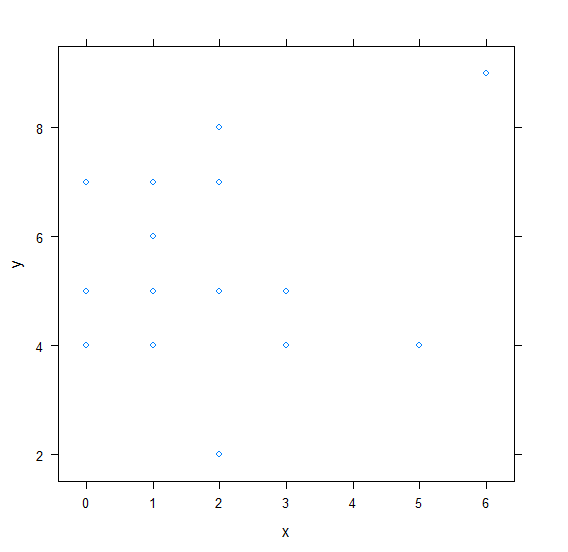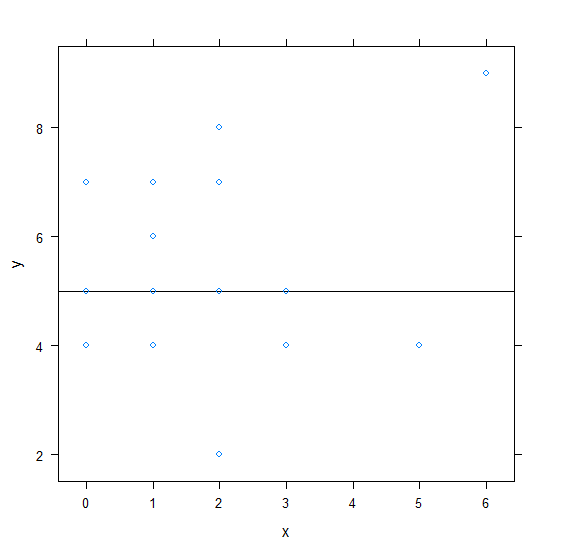# How to create horizontal line in xyplot in R?

To create horizontal line in xyplot, we can use abline function.

For Example, if we have a data frame called df that contains two columns say X and Y and we want to create a scatterplot between X and Y using xyplot with a horizontal line at Y = 2 then we can use the command given below −

xyplot(Y~X,df,abline=c(h=2))

## Example

Following snippet creates a sample data frame −

x<-rpois(20,2)
y<-rpois(20,5)
df<-data.frame(x,y)
df

The following dataframe is created

  x y
1  5 4
2  3 5
3  2 8
4  2 5
5  1 7
6  1 7
7  2 5
8  1 5
9  3 4
10 2 2
11 0 5
12 1 4
13 1 6
14 6 9
15 0 4
16 2 7
17 0 7
18 2 2
19 1 7
20 2 5

To load lattice package and create xyplot for x and y stored in df on the above created data frame, add the following code to the above snippet −

x<-rpois(20,2)
y<-rpois(20,5)
df<-data.frame(x,y)
library(lattice)
xyplot(y~x,df)

## Output

If you execute all the above given snippets as a single program, it generates the following Output −To create xyplot for x and y stored in df with horizontal at Y = 5 on the above created data frame, add the following code to the above snippet −

x<-rpois(20,2)
y<-rpois(20,5)
df<-data.frame(x,y)
library(lattice)
xyplot(y~x,df,abline=c(h=5))

## Output

If you execute all the above given snippets as a single program, it generates the following Output −